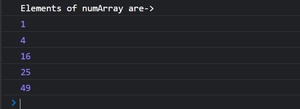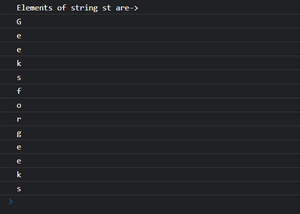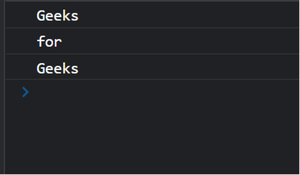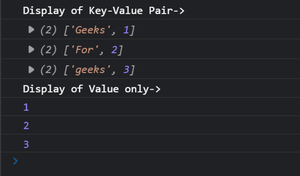Open in App
Not now

# JavaScript for…of Loop

• Last Updated : 07 Nov, 2022

In the previous article of Javascript For..in loop, we can see that we can iterate over only the keys or index of an iterable object like arrays, strings, maps, sets, and so on. But if we try to access the values of the object, then Javascript for..of loop comes into the picture. JavaScript for..of loop was introduced first in Javascript ES6 version.

The for…of loop iterates over the iterable objects (like Array, Map, Set, arguments object, …,etc), invoking a custom iteration hook with statements to be executed for the value of each distinct property.

Syntax:

```for ( variable of iterableObjectName) {
...
}```

Example 1: The following snippet demonstrates the for..of loop over an array.

## HTML

 `<``script``>`` ` `    ``// Array of numbers``    ``let numArray = [1, 4, 16, 25, 49];`` ` `    ``console.log("Elements of numArray are->");`` ` `    ``// for...of Loop``    ``for(let value of numArray){``        ``console.log(value);``    ``}```

Output:Example 2: The following snippet demonstrates the for..of loop over a string.

## HTML

 `<``script``>`` ` `    ``// String object``    ``let st = "Geeksforgeeks";`` ` `    ``// for..of loop``    ``console.log("Elements of string st are->");``    ``for(let value of st){``        ``console.log(value);``    ``}```

Output:Example 3: The following code demonstrates the for..of loop over an argument object.

JavaScript arguments is an object which is local to a function.  It acts as a local variable that is available with all functions by default except arrow functions in JavaScript.

## HTML

 `<``script``>`` ` `    ``// Iterating over argument objects``    ``function Geeks(){``        ``for(let value of arguments){``            ``console.log(value);``        ``}``    ``}`` ` `    ``// Iterating over all arguments passed``    ``// through the function``    ``Geeks("Geeks","for","Geeks");```

Output:Example 4: The following code demonstrates the for..of loop over an argument objects

## HTML

 `<``script``>`` ` `    ``// Map is an object that takes key-value pair as input``    ``let mapObject = new Map([``        ``["Geeks", 1],``        ``["For", 2],``        ``["geeks", 3],``    ``]);`` ` `    ``console.log("Display of Key-Value Pair->")``    ``for (const entry of mapObject) {``        ``console.log(entry);``    ``}``  ` `    ``console.log("Display of Value only->")``    ``for (const [key, value] of mapObject) {``        ``console.log(value);``    ``}```

Output:My Personal Notes arrow_drop_up